#### Notation

For a stratified clustered sample design, observations are represented by an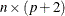matrix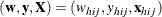where

•denotes the sampling weight vector

•denotes the dependent variable

•denotes the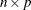design matrix. (When an effect contains only classification variables, the columns ofthat correspond this effect contain only 0s and 1s; no reparameterization is made.)

•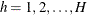is the stratum index

•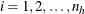is the cluster index within stratum h

•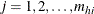is the unit index within cluster i of stratum h

• p is the total number of parameters (including an intercept if the INTERCEPT effect is included in the MODEL statement)

•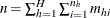is the total number of observations in the sample

Also,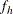denotes the sampling rate for stratum h. You can use the TOTAL= or RATE= option to input population totals or sampling rates. See the section Specification of Population Totals and Sampling Rates for details. If you input stratum totals, PROC SURVEYREG computesas the ratio of the stratum sample size to the stratum total. If you input stratum sampling rates, PROC SURVEYREG uses these values directly for. If you do not specify the TOTAL= or RATE= option, then the procedure assumes that the stratum sampling ratesare negligible, and a finite population correction is not used when computing variances.